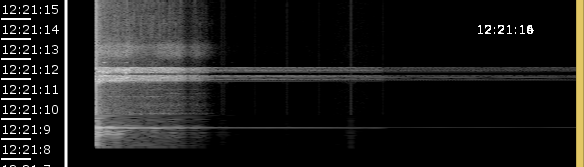#### Howdy, Stranger!

We are about to switch to a new forum software. Until then we have removed the registration on this forum.

# I want the sound representing points to be vertical (See description)

edited May 2017

I have modified a lot of code, added them up and came up with this..Here the sound representing points are presented in horizontal ..I want these points to be put vertical..But with this code I cant get my logic right..Any help?TIA..

``````import ddf.minim.analysis.*;
import ddf.minim.*;
import java.text.SimpleDateFormat;
import java.util.Calendar;
import java.util.List;

Minim minim;
AudioInput in;
FFT fft;
AudioPlayer song;
String str="X coordinate= || Y cordinate=";
ArrayList<String> times = new ArrayList();

// Configuration: spectrogram size (pixels)
int colmax = 500;
int rowmax = 250;
// Sample rate
float sampleRate = 22050;
// buffer size (= FFT size, must be power of 2)
int bufferSize = 1024;

// Variables
int[][] sgram = new int[rowmax][colmax];
int col;
int row;
int leftedge;
int topedge;
int window_len = bufferSize;
String timeHoover="";

PFont mono;

void setup()
{
size(580, 250, P3D);
textMode(SCREEN);
textFont(createFont("SanSerif", 12));

minim = new Minim(this);
song.loop();
// setup audio input
//in = minim.getLineIn(Minim.MONO, bufferSize, sampleRate);

fft = new FFT(song.bufferSize(), song.sampleRate());

// suppress windowing inside FFT - we'll do it ourselves
fft.window(FFT.NONE);
}

void draw()
{
background(0);
stroke(255);
strokeWeight(3);
line(70, 0, 70, height);

//song.play();
// grab the input samples
float[] samples = song.mix.toArray();
// apply windowing
for (int i = 0; i < samples.length/2; ++i) {
// Calculate & apply window symmetrically around center point
// Hanning (raised cosine) window
float winval = (float)(0.5+0.5*Math.cos(Math.PI*(float)i/(float)(window_len/2)));
if (i > window_len/2)  winval = 0;
samples[samples.length/2 - i] *= winval;
samples[samples.length/2 + i] *= winval;
}
// zero out first point (not touched by odd-length window)
samples = 0;

// perform a forward FFT on the samples in the input buffer
fft.forward(samples);

// fill in the new column of spectral values
for (int i = 0; i < colmax /* fft.specSize() */; i++) {
sgram[row][i] = (int)Math.round(Math.max(0, 2*20*Math.log10(1000*fft.getBand(i))));

}

// next time will be the next column
row = row+1;
// wrap back to the first column when we get to the end
if (row == rowmax) {
row = 0;
}

// Draw points.
// leftedge is the column in the ring-filled array that is drawn at the extreme left
// start from there, and draw to the end of the array

for (int i= 0; i<colmax; i++)
{
for (int j=0; j<row; j++)
{
stroke(sgram[j][i]);
point(100+i,j);

}
}

//==============timePrinting
mono = createFont("Verdana", 12);
textFont(mono);
if ( !times.get(0).equals("" + hour() + ":" + minute() + ":" + second())) {
times.add(0, "" + hour() + ":" + minute() + ":" + second());
}
// println(times.size());
while (times.size() > 15) {
times.remove(15);
}
for ( int i = 0; i < times.size(); i++) {
text(times.get(i), 5,  20* i);

if (times.get(i).length()>0)
{
//timeHoover= times.get(i);
stroke(255);
strokeWeight(2);
line(5,20*i+5,35,20*i+5);
}

}

text(timeHoover,480,40);
// Report window size
//text(str, 8, 10);
}

void mousePressed()
{
str="X cordinate = ";
str += String.valueOf(mouseX);
str+=" || Y cordinate = ";
str+=String.valueOf(mouseY);
}

void mouseDragged() {
// map mouse position within window to -1..1
float proportion = map(mouseX, 0, colmax, -1, 0);
// convert to window length, log scale, 2^8 range
window_len = (int)Math.round(Math.pow(2.0, 4.0*proportion)*(float)bufferSize);
text("asdffs",50,50);
}

void mouseMoved()
{

int y = mouseY;
int pos= mouseY/20;
if (pos<times.size())
{
timeHoover = times.get(pos);
text(timeHoover,480,40);
}
}

void stop()
{
// always close Minim audio classes when you finish with them
in.close();
minim.stop();
super.stop();
}
``````

And this is the representation:Tagged:

• (See description)

argh.

just rotate it in the draw. rotate(-HALF_PI) should do it. you might need a translate in there as well, to move it back into the drawable window.

you'll need to change size as well.

• edited May 2017

I dont get it actually about translation #:-S @koogs

• if you have a square with one corner on the origin (o) like this

``````  o 1 2
3 4 5
``````

and rotate it 90 degrees

``````3o
41
52
``````

so that's now left of the origin. and if the origin is the upper left hand corner then the entire shape is now off the screen. you need to translate is to get it back on the screen

• I dont get it actually about translation